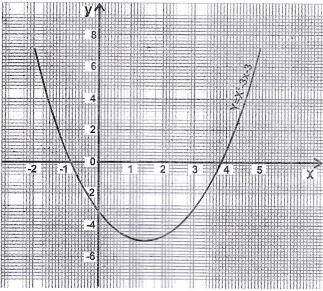## Mathematics 2018 Past Questions | JAMB

Study the following Mathematics past questions and answers for JAMBWAEC  NECO and Post-JAMB. Get prepared with official past questions and answers for upcoming examinations.

31.  A man stands on a tree 150cm high and sees a boat at an angle of depression of 74°. Find the distance of the boat from the base of the tree.

• A. 52cm
• B. 43cm
• C. 40cm
• D. 15cm

32.  Integrate the expression 6x$$^2$$ – 2x + 1

• A. 3x$$^3$$ – 2x$$^2$$ + x + c
• B. 2x$$^3$$ – x$$^2$$ + x + c
• C. 2x$$^3$$ – 3x$$^2$$ + c
• D. x$$^3$$ + x$$^2$$ – x + c

33.  In how many ways can the letters LEADER be arranged?

• A. 72
• B. 144
• C. 360
• D. 720

34.In the figure below, /MX/ = 8cm, /XN/ = 12cm, /NZ/ = 4cm and ∠ XMN = ∠ XZY. Calculate /YM/

• A. 32cm
• B. 24 cm
• C. 16 cm
• D. 12 cm

35. Express 495g as a percentage of 16.5kg

• A. 3%
• B. 3 1/3%
• C. 15%
• D. 30%

36. Evaluate (2√3 – 4) (2√3 + 4)

• A. -4
• B. -2
• C. 2
• D. 4

37. Find the equation of the tangent at the point (2, 0) to the curve y = x² – 2x

• A. y = 2x – 4
• B. y = 2x + 4
• C. y = 2x – 2
• D. y = 2x + 2

38.What is the minimum value of the graph?

• A. -5.3
• B. 0.5
• C. 3
• D. 8

39. Evaluate $$(\frac{6}{0.32} \div \frac{2}{0.084})^{-1}$$ correct to 1 decimal place.

• A. 1.3
• B. 2.5
• C. 4.6
• D. 3.2

40.  Evaluate log$$_2$$ 8 – log$$_3$$ $$\frac{1}{9}$$

• A. -1 1$$\frac{1}{2}$$
• B. -1
• C. 1
• D. 5

## All Subjects

Subscribe
Notify of